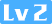1 手写ArrayList核心源码九路528 1 1

## 手写ArrayList核心源码

public class QList<T> {
//默认的数组的大小
private final int DEFAULT_LIST_SIZE = 8;

//存放数据的地方
private Object[] mData;

//下一个可以存放数据的当前数组的索引
private int mSize;

......
}    

public class QArrayList<T> {
//默认的数组的大小
private final int DEFAULT_LIST_SIZE = 8;

//存放数据的地方
private Object[] mData;

//下一个可以存放数据的当前数组的索引
private int mSize;

public QArrayList() {
//new 一个数组，用来存放
mData = new Object[DEFAULT_LIST_SIZE];

//下一个可以存放数据的当前数组的索引为0
mSize = 0;
}

public QArrayList(int capacity){
if(capacity <= 0 || capacity > Integer.MAX_VALUE){
throw new RuntimeException("invalid capacity");
}

mData = new Object[capacity];
mSize = 0;
}

//返回当时数组的已经存放了多少个元素
public int size() {
return mSize;
}

//返回数组的总大小，其实这个接口没有必要对外提供，这里我们只是为了演示用
public int capacity() {
return mData.length;
}

//添加一个元素
//规定不允许添加一个空元素
if(e == null){
return;
}

//如果当前数组已经满了，扩容为原来数组的2倍
if (mSize >= mData.length) {

//扩容
resize();
}

//将添加的元素添加到数组中
mData[mSize] = e;

//同时 mSize++ 指向下一个可以存放数据的位置
mSize++;
}

//获取指定位置的元素，如果position不合法，直接抛出异常
//这样做是有必要的，我们提供的是一个库
// 直接抛出异常让使用知道用错了，没有必要 return null
// 因为这是个库，不是业务，就算return null，也是业务层的事
public T get(int position) {
if (position < 0 || position >= mData.length) {
throw new RuntimeException("position is invalid");
}

// position 大于 mSize 也没有关系，因为也是返回null，证明没有获取到
return (T) mData[position];
}

//删除指定位置的元素
public T remove(int position) {
//和上面一样，位置不合法直接抛出异常
if (position < 0 || position >= mData.length) {
throw new RuntimeException("position is invalid");
}

//把当前要删除的元素保存下来，最后返回要删除的元素
T e = (T) mData[position];

//删除后，把后面的所有元素都往前移位
for (int i = position + 1; i < mData.length; i++) {
mData[i - 1] = mData[i];
}

//别忘了 mSize 要 --
mSize--;

//返回删除的元素
return e;
}

//删除指定的元素
public boolean remove(T e) {
//因为数组可能没有满，如果删除的是null，没有必要，我们不允许
if (e == null) {
return false;
}

//找到删除元素的位置
int position = -1;
for (int i = 0; i < mData.length; i++) {
if (e == mData[i] || e.equals(mData[i])) {
position = i;
break;
}
}

//没有找到就返回
if (position == -1) {
return false;
}

//删除
return remove(position) != null;
}

//扩容,我们都以2倍的容量扩容
private void resize() {
Object[] old = mData;
mData = new Object[mData.length * 2];
for (int i = 0; i < old.length; i++) {
mData[i] = old[i];
}

old = null;
}
}


    public static void main(String[] args) {
QArrayList<String> list = new QArrayList<>();

System.out.println("list.get(2)=" + list.get(2));
System.out.println("list.size()=" + list.size());
for (int i = 0; i < list.size(); i++) {
System.out.println("list.get(" + i + ") = " + list.get(i));
}

System.out.println("=======================");
System.out.println("演示删除操作");
list.remove("jim");

for (int i = 0; i < list.size(); i++) {
System.out.println("list.get(" + i + ") = " + list.get(i));
}
}


 public static void main(String[] args) {
//新建一个只有2个元素的数组
QArrayList<String> list = new QArrayList<>(2);

//打印出扩容后的容量
System.out.println("扩容前 ： list.capacity()=" + list.capacity());

//我们添加了4个元素

//打印出扩容后的容量
System.out.println("扩容后 ： list.capacity()=" + list.capacity());

//打印
for (int i = 0; i < list.size(); i++) {
System.out.println("list.get(" + i + ") = " + list.get(i));
}
}

### 相关推荐

1 手写ArrayList核心源码

List集合
Java的List集合 一、ArrayList 1.插入 java / 在元素序列尾部插入 / public boolean add(E e) { // 1. 检测是否需要扩容 ensureCapacityInternal(size 1); // Increments modCount // 2. 将新元素插入序列尾
Linux下安装jdk

Python中的基本list操作
List是python中的基本数据结构之一，和Java中的ArrayList有些类似，支持动态的元素的增加。list还支持不同类型的元素在一个列表中，List is an Object。 最基本的创建一个列表的方法 myList \'a','b','c'\ 在python中list也是对象，所以他也有方法和属性，在ptython解释器中 使用h

ArrayList是List接口的实现类，它是支持根据需要而动态增长的数组。java中标准数组是定长的，在数组被创建之后，它们不能被加长或缩短。这就意味着在创建数组时需要知道数组的所需长度，但有时我们需要动态程序中获取数组长度。ArrayList就是为此而生的,但是它不是线程安全的，外ArrayList按照插入的顺序来存放数据 ①ArrayList扩容发生
java中List数组遍历删除
List数组遍历删除 环境 jdk8 junit 单元测试 正解java// 正解1, jdk自带的addAll方法 @Test public void test18() { String strs {"12","34","56","78","90"}; List<String list Ar
ArrayList底层

[C#]ArrayList、string、string[]之间的转换
1、ArrarList 转换为 string ：　　ArrayList list new ArrayList();　　list.Add("aaa");　　list.Add("bbb");　　string arrString (string)list.ToArray(typeof( string)) ;2、string 转换
Java TreeMultiSet-为什么要开发这个数据结构？？？